Examples for 7th grade (seventh) - page 69

1. Photo enlarge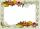Mark wants to enlarge a 4-inch by 6-inch photo so that it has a height of 15inches. Use a ratio table to determine the new width of the photo.
2. CandyPeter had a sachet of candy. He wanted to share with his friends. If he gave them 30 candies, he would have 62 candies. If he gave them 40 candies, he would miss 8 candies. How many friends did Peter have?
3. Unknown numbersThe sum of two consecutive natural numbers and their triple is 92. Find these numbers.
4. Clubhouse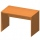There were only chairs and table in the clubhouse. Each chair had four legs, and the table was triple. Scouts came to the clubhouse. Everyone sat on their chair, two chairs were left unoccupied, and the number of legs in the room was 101. How many chairs w
5. Two cuboidsFind the volume of cuboidal box whose one edge is: a) 1.4m and b) 2.1dm
6. Five pumpsThe water tank is filled with two pumps in 48 minutes. How long would it take to fill it with 5 same pumps?
7. Soda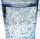A pack of 12 sodas cost 5.04. What is the unit rate in dollars per soda?
8. Bathroom 2A bathroom is 2.4 meters long and 1.8 meters wide. How many square tiles 1 dm on each side are to be used to cover it?
9. Unknown numberI think the number - its sixth is 3 smaller than its third.
10. RainfallHow many liters of water did fell in a 32m long and 8m wide garden, if 8mm of rain fell?
11. SuzanSusan's age will be after 12 years four times as much as twelve years ago. How old is Susan now?
12. Trapezium basesFind the trapezium height if a = 8 cm and c = 4 cm if its content 21 square centimeters.
13. Trapezoid ABCDABDC is a trapezoid in which AB and CD are parallel sides measuring 6 and 9 respectively. Angles ABC and BCD are both right angles. Find the length of segment BD.
14. Cubes into cuboidHow many 12 centimeter cubes fit into the block (cuboid) with 6dm, 8,4dm and 4,8?
15. PearsThere were pears in the basket, I took two-fifths of them, and left six in the basket. How many pears did I take?
16. Summands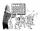Find two summands of the number 42, so that its product is minimized.
17. Square area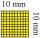Complete the table and then draw each square. Provide exact lengths. Describe any problems you have. Side Length Area .
18. Bicycle gears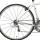The toothed wheel on the bicycle pedal has 40 teeth, the wheel on the rear wheel has only 16 teeth. How many times does the rear wheel turn if the pedals rotate 50 times?
19. Warmer weatherThis morning it was -6 °C. What temperature did the thermometer show yesterday if it was three times warmer?
20. Surface area of the topA cylinder is three times as high as it is wide. The length of the cylinder’s diagonal is 20 cm. Find the surface area of the top of the cylinder.

Do you have an interesting mathematical example that you can't solve it? Enter it, and we can try to solve it.

To this e-mail address, we will reply solution; solved examples are also published here. Please enter e-mail correctly and check whether you don't have a full mailbox.